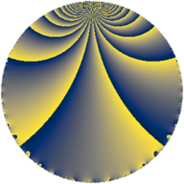# Properties

 Label 63.2.pLevel $63$ Weight $2$ Character orbit 63.p Rep. character $\chi_{63}(17,\cdot)$ Character field $\Q(\zeta_{6})$ Dimension $4$ Newform subspaces $1$ Sturm bound $16$ Trace bound $0$

# Related objects

## Defining parameters

 Level: $$N$$ $$=$$ $$63 = 3^{2} \cdot 7$$ Weight: $$k$$ $$=$$ $$2$$ Character orbit: $$[\chi]$$ $$=$$ 63.p (of order $$6$$ and degree $$2$$) Character conductor: $$\operatorname{cond}(\chi)$$ $$=$$ $$21$$ Character field: $$\Q(\zeta_{6})$$ Newform subspaces: $$1$$ Sturm bound: $$16$$ Trace bound: $$0$$

## Dimensions

The following table gives the dimensions of various subspaces of $$M_{2}(63, [\chi])$$.

Total New Old
Modular forms 24 4 20
Cusp forms 8 4 4
Eisenstein series 16 0 16

## Trace form

 $$4 q - 2 q^{7} + O(q^{10})$$ $$4 q - 2 q^{7} - 12 q^{10} + 8 q^{16} + 6 q^{19} - 8 q^{22} - 2 q^{25} + 6 q^{31} + 2 q^{37} + 24 q^{40} - 4 q^{43} + 16 q^{46} - 26 q^{49} - 8 q^{58} - 12 q^{61} - 32 q^{64} - 22 q^{67} + 24 q^{70} + 6 q^{73} - 10 q^{79} - 36 q^{82} + 48 q^{85} + 8 q^{88} + 54 q^{91} + 60 q^{94} + O(q^{100})$$

## Decomposition of $$S_{2}^{\mathrm{new}}(63, [\chi])$$ into newform subspaces

Label Dim $A$ Field CM Traces $q$-expansion
$a_{2}$ $a_{3}$ $a_{5}$ $a_{7}$
63.2.p.a $4$ $0.503$ $$\Q(\sqrt{-2}, \sqrt{-3})$$ None $$0$$ $$0$$ $$0$$ $$-2$$ $$q+\beta _{1}q^{2}+(-\beta _{1}+2\beta _{3})q^{5}+(1-3\beta _{2}+\cdots)q^{7}+\cdots$$

## Decomposition of $$S_{2}^{\mathrm{old}}(63, [\chi])$$ into lower level spaces

$$S_{2}^{\mathrm{old}}(63, [\chi]) \cong$$ $$S_{2}^{\mathrm{new}}(21, [\chi])$$$$^{\oplus 2}$$